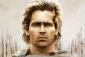# How can i compute the height of the ice cube to be put in a conical tank when it turns into a liquid

2 posts / 0 new
kullboys
How can i compute the height of the ice cube to be put in a conical tank when it turns into a liquid

Hello everyone
How can i compute the height of the ice cube to be put in a conical tank when it turns into a liquid (water). Given with a height of the conical tank and its radius and the volume of the cube.
Thanks

Tags:
AlexanderThis is interesting, the question however is not clear, but this is how I understand it, and I hope I am correct: What is the depth of the water inside the cone when the ice melted into water?

If we will not consider the expansion of water when it turned into ice, we will simply equate the volume of the ice cube and the volume of water inside the cone. We can do ratio and proportion to express the radius of water surface in terms of the depth of water and we will have an equation of depth of water alone as the unknown. This way, we solve the problem.

If Physics will come into play, we need to consider the volumetric expansion of water to ice, I think the coefficient of that expansion is constant. When ice melts into water, the volume of water is a little less than the volume of ice cube.

• Mathematics inside the configured delimiters is rendered by MathJax. The default math delimiters are $$...$$ and $...$ for displayed mathematics, and $...$ and $...$ for in-line mathematics.